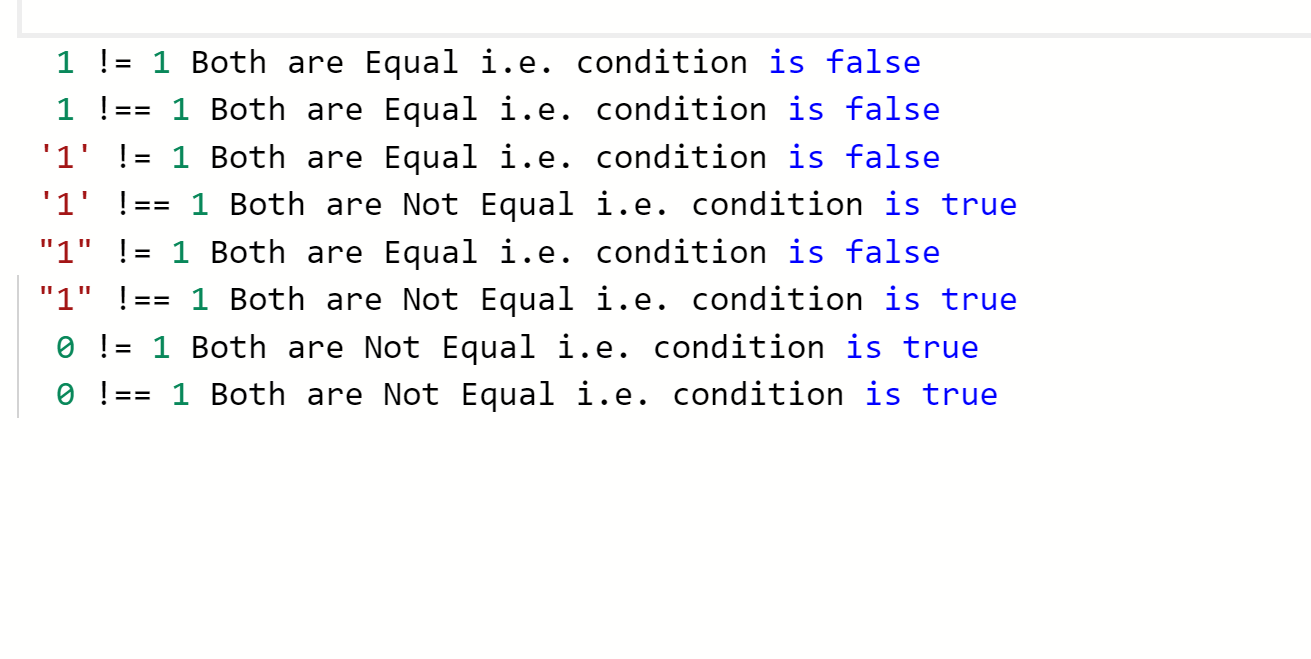# Difference between != and !== operator in JavaScript

• Last Updated : 07 Aug, 2019
• != operator
• The inequality operator (!=) is the logical opposite of the equality operator. It means “Not Equal” and returns true where equality would return false and vice versa.
• Like the equality operator, the inequality operator will convert data types of values while comparing.
• For example 1 != ‘1’ will return false since data type conversion take place so 1 and ‘1’ are considered equal.
• !== operator
• The strict inequality operator (!==) is the logical opposite of the strict equality operator. It means “Strictly Not Equal” and returns true where strict equality would return false and vice versa.
• Strict inequality will not convert data types.
• For example 1 !== ‘1’ will return true since 1 is an integer and ‘1’ is a character and no data type conversion take place.

Example:

 ``

Output:My Personal Notes arrow_drop_up